## Math 480 Course Notes -- May 30, 1996

### Stochastic processes continuous in time, discrete in space

The second kind of stochastic process we will consider is a process X(t) where the time t is a real-valued deterministic variable and at each time t, the sample space S of the random variable X(t) is a (finite or infinite) discrete set. In such a case, a state X of the entire process is described as a sequence of jump times (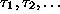) and a sequence of values of X (elements of the common discrete state space)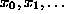. These describe a "path" of the system through S given by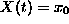for 0<t<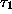,for<t<, etc... Because X moves by a sequence of jumps, these processes are often called "jump" processes.

Thus, to describe the stochastic process, we need, for each x in S, a continuous random variablethat describes how long it will be before the process (starting at X=x) will jump, and a set of discrete random variables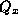that describe the probabilities of jumping from x to other states when the jump occurs. We will letbe the probability that the state starting at x will jump to y (when it jumps), so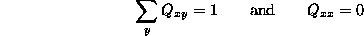We also define the functionto be the probability that the process starting at X=x has already jumped at time t, i.e.,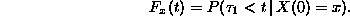All this is defined so that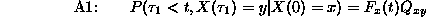(this is an independence assumption: where we jump to is independent of when we jump.

Instead of writing, we will begin to write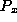(...).

We will make the usual stationarity and Markov assumptions as follows: We assume first that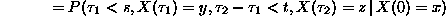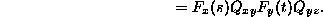It will be convenient to define the quantityto be the probability that X(t)=y, given that X(0)=0, i.e.,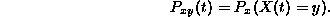Then the Markov assumption is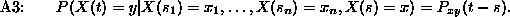The three assumptions A1, A2, A3 will enable us to derive a (possibly infinite) system of differential equations for the probability functions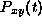for x and y in S. First, the stationarity assumption A1 tells us thatThis means that the probability of having to wait at least one minute more before the process jumps is independent of how long we have waited already, i.e., we can take any time to be t=0 and the rules governing the process will not change. (From a group-theoretic point of view this means that the process is invariant under time translation). Using the formula for conditional probability (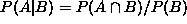), the fact that the event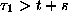is a subset of the event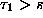, we translate this equation into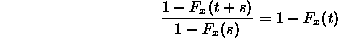This implies that the function 1-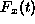is a multiplicative function, i.e., it must be an exponential function. It is decreasing sincerepresents the probability that something happens before time t, and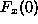=0, so we must have that 1-=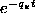for some positive number. In other words,

•is exponentially distributed with probability density function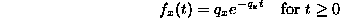To check this, note that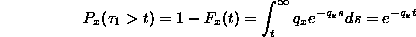so the two ways of computingagree.

The next consequence of our stationarity and Morkov assumptions are the Chapman-Kolmorgorov equations in this setting:They say that to calculate the probability of going from state x to state y in time t+s, one can sum up all the ways of going from x to y via any z at the intermediate time t. This is an obvious consistency equation, but it will prove very useful later on.

Now we can begin the calculations that will produce our system of differential equations fod. The first step is to expressbased on the time of the first jump from x. The result we intend to prove is the following:As intimidating as this formula looks, it can be understood in a fairly intuitive way. We take it a term at a time:

•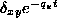: This term is the probability that the first jump hasn't happened yet, i.e., that the state has remained x from the start. That is why the Kronecker delta symbol is there (recall that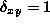if x=y and =0 if x is different from y). This term is zero unless x=y, in which case it gives the probability that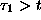, i.e., that no jump has occurred yet.

• The integral term can be best understood as a "double sum", and then by using the Chapman Kolmorgorov equation. For any s between 0 and t, the definition oftells us that the probability that the first jump occurs between time s and time s+ds is (approximately)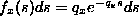(where the error in the approximation goes to zero faster than ds as ds goes to zero). Therefore, the probability that for z different from x, the first jump occurs between time s and time s+ds and the jump goes from x to z is (approximately). Based on this, we see that the probability that the first jump occurs between time s and time s+ds, and the jump goes from x to z, and in the intervening time (between s and t) the process goes from z to y is (approximately)To account for all of the ways of going from x to y in time t, we need to add up these probabilities over all possible times s of the first jump (this gives an integral with respect to ds in the limit as ds goes to 0), and over all possible results z different from x of the first jump. This reasoning yields the second term of the formula.

We can make a change of variables in the integral term of the formula: let s be replaced by t-s. Note that this will transform ds into -ds, but it will also reverse the limits of integration. The result of this change of variables is (after taking out factors from the sum and integral that depend neither upon z nor upon s) :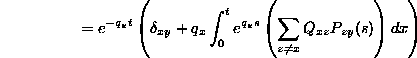Nowis a continuous function of t because it is defined as the product of a continuous function with a constant plus a constant times the integral of a bounded function of t (the integrand is bounded on any bounded t interval). But onceis continuous, we can differentiate both sides of the last equation with respect to t and show thatis also differentiable.

We take this derivative (and take note of the fact that when we differentiate the first factor we just get a constant times what we started with) using the fundamental theorem of calculus to arrive at: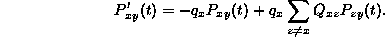A special case of this differential equation occurs when t=0. Note if we know that X(0)=x, then it must be true that. This observation enables us to write: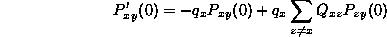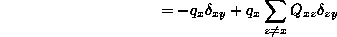One last bit of notation! Let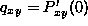. So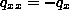and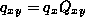if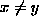. (Note that this implies thatso the sum of the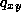as y ranges over all of S is 0. the constantsare called the infinitesimal parameters of the stochastic process.

Given this notation, we can rewrite our equation for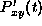given above as follows: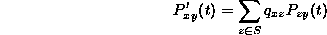for all t>0. This system of differential equations is called the backward equation of the process.

Another system of differential equations we can derive is the forward equation of the process. To do this, we differentiate both sides of the Chapman Kolmogorov equation with respect to s and get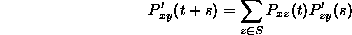If we set s=0 in this equation and use the definition of, we get the forward equation: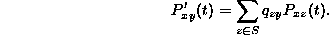Next time: We will use the forward equation to show the relationship between the exponential and Poisson distributions, and examine other examples of jump processes.

### Homework problems:

1. Retry the problem of calculating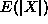andfor one-dimensional random walk on the line.

2. What are the eigenvalues of the transition matrix for the random walk on the circle problem (i.e., the sharing problem)? How are these related to the rate of convergence to the limiting distribution?

Dennis DeTurck
Thu May 30 16:47:29 EDT 1996